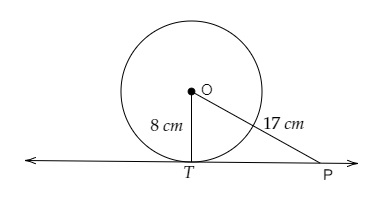# If PT is a tangent at T to a circle whose centre is O and $OP = 17\ cm$, $OT = 8\ cm$. Find the length of the tangent segment PT.

Given:

PT is a tangent at T to a circle whose centre is O and $OP = 17\ cm$, $OT = 8\ cm$.

To do:

We have to find the length of the tangent segment PT.

Solution:$OP = 17\ cm$ and $OT = 8\ cm$

$PT$ is the tangent to the circle at $T$.

$OT\ perp\ PT$

In right angled triangle $OTP$,

By Pythagoras theorem,

$OP^2= OT^2+ TP^2$

$17^2 = 8^2 + TP^2$

$TP^2= 289 - 64$

$= 225$

$= 15^2$

Therefore,

$TP = 15\ cm$

The length of the tangent segment PT is 15 cm.

Updated on: 10-Oct-2022

17 Views